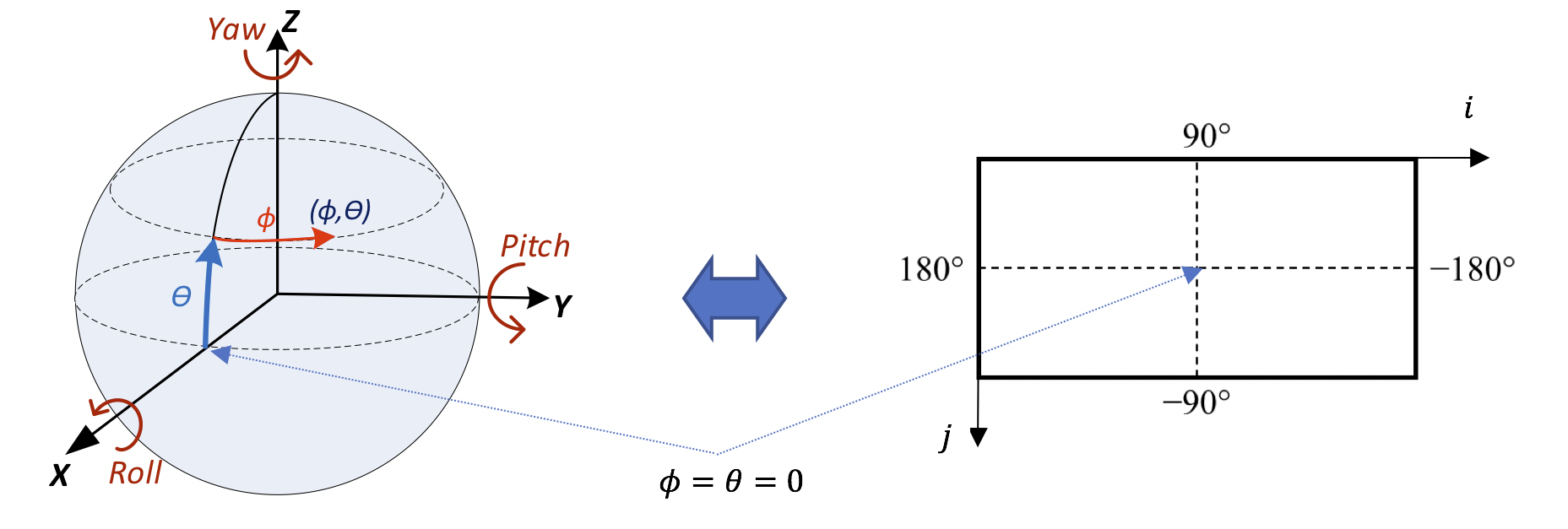## A.2.3.2 Equirectangular Projection (ERP)

26.1183GPPRelease 17TSVirtual Reality (VR) profiles for streaming applications

Equirectangular mapping is the most commonly used mapping from spherical video to a 2D texture signal. The mapping is bijective, i.e. it may be expressed in both directions and is illustrated in Figure A.2.Figure A.2: Mapping of spherical video to a 2D texture signal

ERP has only one face and the face index f for ERP is always set to 0. The sphere coordinates (ϕ, θ) for a sample location (i, j), in degrees, are given by the following equations:

ϕ = (0.5 – i/pictureWidth)*360

θ = (0.5 – j/pictureHeight)*180

Finally, (X, Y, Z) can be calculated from the equations given above.